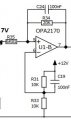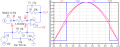# non inverting OP-Amp used as comparator

#### gerdomerdo

Joined Apr 2, 2021
9
In the schematic below:The circuit is behaving as a comparator. When V+>V- the output is at 12V. When V+<V- the output is 0. (the positive pin power supply of the op-amp is connected to 12V and the nagative pin power supply is connected to ground).

The problem is when I try to do the math derivation to justify the behavior of the op-amp, I don't get what that comparator effect.
Here is the math derivation:
At V- (pin6), we have 6V because of the voltage divider R33/(R31+R33). Which means that we should get 6V at the V+(pin 5).
To make it simple, no currents flow in pins 5 and 6 (in reality there are some pAmps). the current flowing in R35 is (7V-6V)/R35=0.14mA.
then this current is flowing also in R34, since V5 is at 6V, the voltage at pin 7 (the output) is 2.93V (20kR*0.14mA). In LTspice I got at pin 7 12V.
I would like to know how I can justify the behavior of such circuit.

#### LvW

Joined Jun 13, 2013
1,486
.......................
At V- (pin6), we have 6V because of the voltage divider R33/(R31+R33). Which means that we should get 6V at the V+(pin 5).
Why do you think that there are also 6V at pin 5 ?

#### gerdomerdo

Joined Apr 2, 2021
9
@LvW , that's how an amplifier operates when there is a feedback, it tries to maintain the V+ and V- at the same potential

#### Danko

Joined Nov 22, 2017
1,405

#### LvW

Joined Jun 13, 2013
1,486
@LvW , that's how an amplifier operates when there is a feedback, it tries to maintain the V+ and V- at the same potential
Yes - that is correct, but for NEGATIVE feedback only. Positive feedback causes saturation and the mentioned rule does NOT apply anymore.

#### gerdomerdo

Joined Apr 2, 2021
9
Yes - that is correct, but for NEGATIVE feedback only. Positive feedback causes saturation and the mentioned rule does NOT apply anymore.
Ah okay , but can we prove it mathemtically ? I mean how can we reach the saturation in this case.

#### LvW

Joined Jun 13, 2013
1,486
Ah okay , but can we prove it mathemtically ? I mean how can we reach the saturation in this case.
Saturation means that the opamp output reaches its limits (power supply rail). The amplifier has left his linear range. Because of the very large dc gain and due to missing negative feedback), some tenth of µVolts between the inputs are enough to reach this state .
Question: Are you using dual or single supply for the opamp?

#### gerdomerdo

Joined Apr 2, 2021
9
Saturation means that the opamp output reaches its limits (power supply rail). The amplifier has left his linear range. Because of the very large dc gain and due to missing negative feedback), some tenth of µVolts between the inputs are enough to reach this state .
Question: Are you using dual or single supply for the opamp?
I am using a single supply, I just want to know what is the voltahe at the pin 5 (+). (I know that it's greater than V-), but what is the exact value, so that we can have that voltage difference at the inputs.

#### LvW

Joined Jun 13, 2013
1,486
I am using a single supply, I just want to know what is the voltahe at the pin 5 (+). (I know that it's greater than V-), but what is the exact value, so that we can have that voltage difference at the inputs.
Voltage at pin 5 (+) under which condition? 7 Volts DC at R35 ?

#### BobTPH

Joined Jun 5, 2013
5,507
The voltage on pin 5 depends on the input voltage and the output voltage only. Write an expression for it in terms of those two voltages.

Bob

#### LvW

Joined Jun 13, 2013
1,486
The voltage on pin 5 depends on the input voltage and the output voltage only. Write an expression for it in terms of those two voltages.
Bob
Yes - thats why I have asked for the input voltage .
Its nothing else than a case for superposition.

#### gerdomerdo

Joined Apr 2, 2021
9
Yes - thats why I have asked for the input voltage .
Its nothing else than a case for superposition.
The DC input voltage is 7V at R35, I still don't see the voltage difference at the inputs

#### Danko

Joined Nov 22, 2017
1,405
Capacitors value is changed:See how works ideal opamp.
Pay attention to R2 value:
R2=(R1/Vref)*(Vth-Vref) this formula is accurate for ideal opamp only.Vth is high going threshold voltage.Last edited:

#### BobTPH

Joined Jun 5, 2013
5,507
Yes - that`s why I have asked for the input voltage .
Its nothing else than a case for superposition.
My post was directed to the TS, I know you can do it

Bob

#### gerdomerdo

Joined Apr 2, 2021
9
My post was directed to the TS, I know you can do it

Bob
I could not do it that's why I asked for help

#### crutschow

Joined Mar 14, 2008
30,460
I could not do it that's why I asked for help
You have two separate voltage divider problems involving two resistors for each divider.
Are you saying you don't know how to do that?

#### gerdomerdo

Joined Apr 2, 2021
9
You have two separate voltage divider problems involving two resistors for each divider.
Are you saying you don't know how to do that?
I am asking about how to calculate the voltage at pin 5, if we consider that we don't know the output voltage we are having two unkown voltages. If it's simple to you explain it to me

#### crutschow

Joined Mar 14, 2008
30,460
The op amp acting as a comparator with positive feedback, so the output has two states, either positive saturation (plus input higher than the negative input) or negative saturation (plus input lower than the negative input).

So you calculate the input voltage difference for both conditions of the output, and see which one leads to the input voltage difference that is correct one for the two possible output voltages.

Make sense?

•gerdomerdo